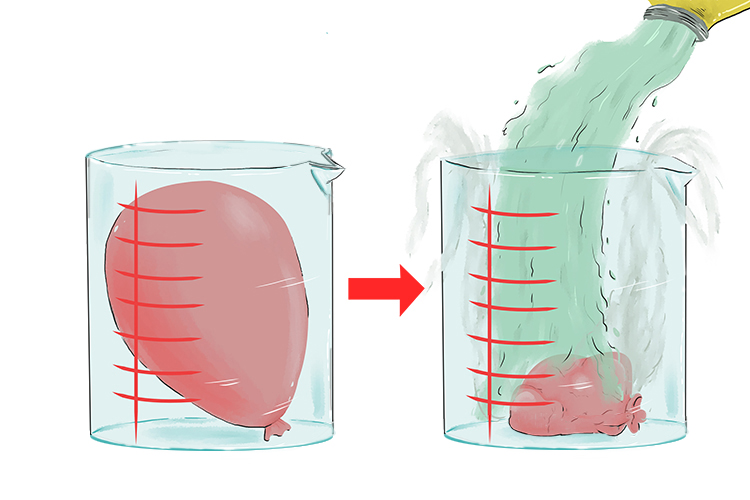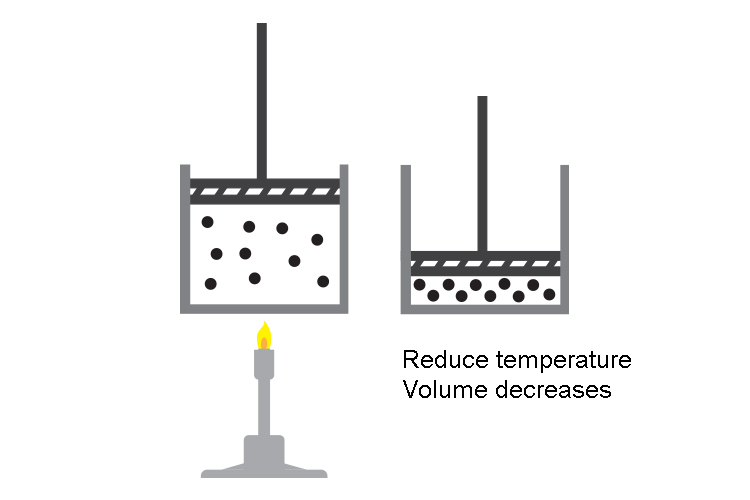# Charles' Law In Use

We know that in Charles' law

V_1/T_1=V_2/T_2

NOTE: Pressure remains constant

Also, if you put a balloon inside a bucket and pour cold liquid nitrogen over the balloon, you can watch it shrink.This is Charles' Law

V_1 and T_1 refer to the volume and temperature before you pour the liquid nitrogen on the balloon and V_2 and T_2 refer to the volume and temperature after you pour the liquid nitrogen on the balloon.

Reduce the temperature of the gas and the volume of the gas reduces too.Reducing the temperature of a gas slows down the molecules and they have less energy (kinetic energy) to hit and push the sides of the balloon. The molecules will exert less force and so the external atmospheric pressure will collapse the balloon.

NOTE 1:

The standard unit used to measure volume is m^3 (metre cubed)

The standard unit used to measure temperature is K (Kelvin)

NOTE 2:

You can use other units for volume such as m^3 or ml as long as you use the same units either side of the equation but you must use Kelvin for temperature.

NOTE 3:

Never forget to convert temperature to Kelvin

Temperature in Kelvin = temperature in degrees Celsius (\ ^(circ)C)+273

You must always use temperature measured in Kelvin in any gas law equation.

Examples

1. A container holds 50.0\ \ml of nitrogen at 25^circC and a pressure of 736\ \mm\ \Hg. What will be its volume if the temperature increases by 35^circC ?

1. A container holds 50.0\ \ml of nitrogen at 25^circC and a pressure of 736\ \mm\ \Hg. What will be its volume if the temperature increases by 35^circC ?

V_1/T_1=V_2/T_2

Therefore V_2=(V_1xxT_2)/T_1

V_1=50\ \ml

V_2=?

T_1=25^circC+273=298K

T_2=25^circC+35^circC+273=333K

Therefore V_2=(50xx333)/298

V_2=55.9\ \ml

2. 568\ \cm^3 of chlorine at 25^circC will occupy what volume at -25^circC while the pressure remains constant?

2. 568\ \cm^3 of chlorine at 25^circC will occupy what volume at -25^circC while the pressure remains constant?

V_1/T_1=V_2/T_2

V_1=568\ \cm^3

V_2=?

T_1=25^circC+273=298K

T_2=-25^circC+273=248K

Therefore V_2=(V_1xxT_2)/T_1

V_2=(568xx248)/298

V_2=473\ \cm^3

3. A sample of hydrogen has an initial temperature of 50^circC. When the temperature is lowered to -5.0^circC, the volume of hydrogen becomes 212\ \cm^3. What was the initial volume of the hydrogen?

3. A sample of hydrogen has an initial temperature of 50^circC. When the temperature is lowered to -5.0^circC, the volume of hydrogen becomes 212\ \cm^3. What was the initial volume of the hydrogen?

V_1/T_1=V_2/T_2

V_1=?

V_2=212\ \cm^3

T_1=50^circC+273=323K

T_2=-5^circC+273=268K

Therefore V_1=(V_2xxT_1)/T_2

V_1=(212xx323)/268

V_1=256\ \cm^3